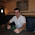### Cracking moment

The cracking moment is the moment corresponding tensile bending stress at which concrete will start to crack. reinforcing steel cross-sectional area is 2% or less of concrete beam cross-section. the area of reinforcing steel is minimal compared to concrete. therefore, it will not cause a major change to concrete beam properties as long as the beam uncracked. bending stress for concrete at distance y from a neutral axis can be calculated using elastic beam theory

f=M.y/I

where
f is the bending stress at distance y
M is the moment applied to the beam
y is the distance from the neutral axis
I is the moment of inertia for beam

concrete modulus of rupture is defined as the bending tensile strength at which concrete start to cracks. ACI provides us with the equation for calculating the modulus of rupture

fr=7.5λ√fc' (in psi)
fr=0.7λ√fc' (in Mpa)
where
λ is equal 1 for normal weight concrete and less than 1 for lightweight concrete
fc' is the compressive strength for concrete

now the tensile bending stress at which concrete start to cracks is known. we can calculate the cracking moment. rearranging previous equation

Mcr=fr.I/yt

where
Mcr is the cracking moment
fr is the modulus of rupture for concrete
I is the moment of inertia for beam
yt is the distance from the neutral axis to extreme fiber in tension

Figure 1

Example: assuming concrete is uncracked for the beam shown in figure no:2. consider dead load plus distributed load of 2 kN/m. fc'=30Mpa
• calculate bending stress at extreme tension fiber
• calculate the cracking moment
unit weigth of concrete equal 24.50 kn/m3 , Length of beam=5 m

I=bh3/12=0.002278 m4

max moment for simply supported beam=(W*L^2)/8=16.56 kn.m

neutral axis located at the center of the beam due to symmetry

therefore, bending stress at etreme tensile fiber located at 0.225 from neutral axis
ft=(16.56*0.225)/0.002278=1,635.89 kn/m2=1.63 Mpa

modulus of rupture=0.7*1*√30=3.83 mpa=3,830kn/m2

Mcr=fr.I/yt=3830*(0.002278)/0.225=38.77 kn.m

Figure 2

More examples

1.Fr =.7 fc^.5
And fc = Mpa
Then how come the unit of fr is still Mpa ? ( Not Mpa^.5)

1.The equation modulusfor of rupture for concrete is taken for ACI (9-10) code. The equation is empirical relationship based on extensive experimental data.